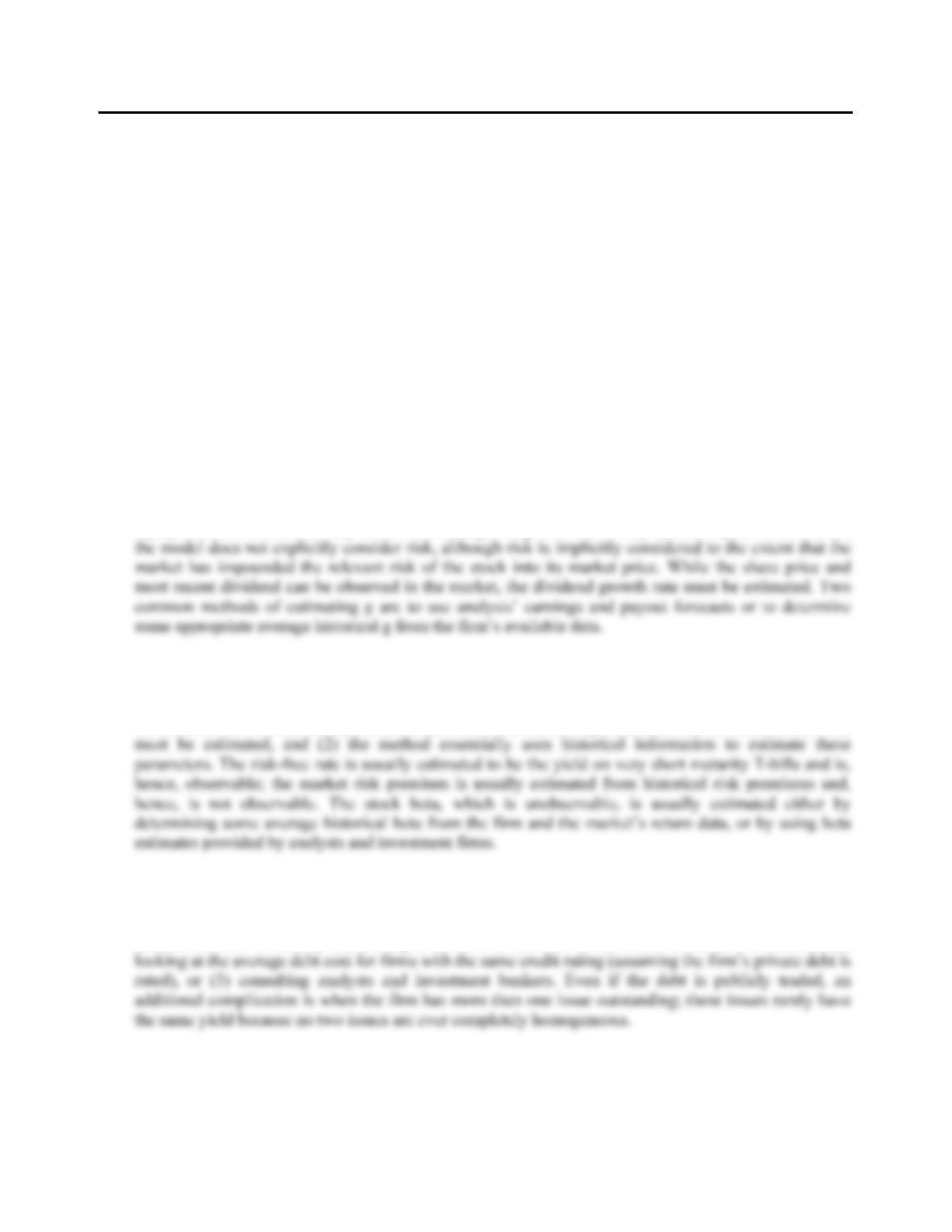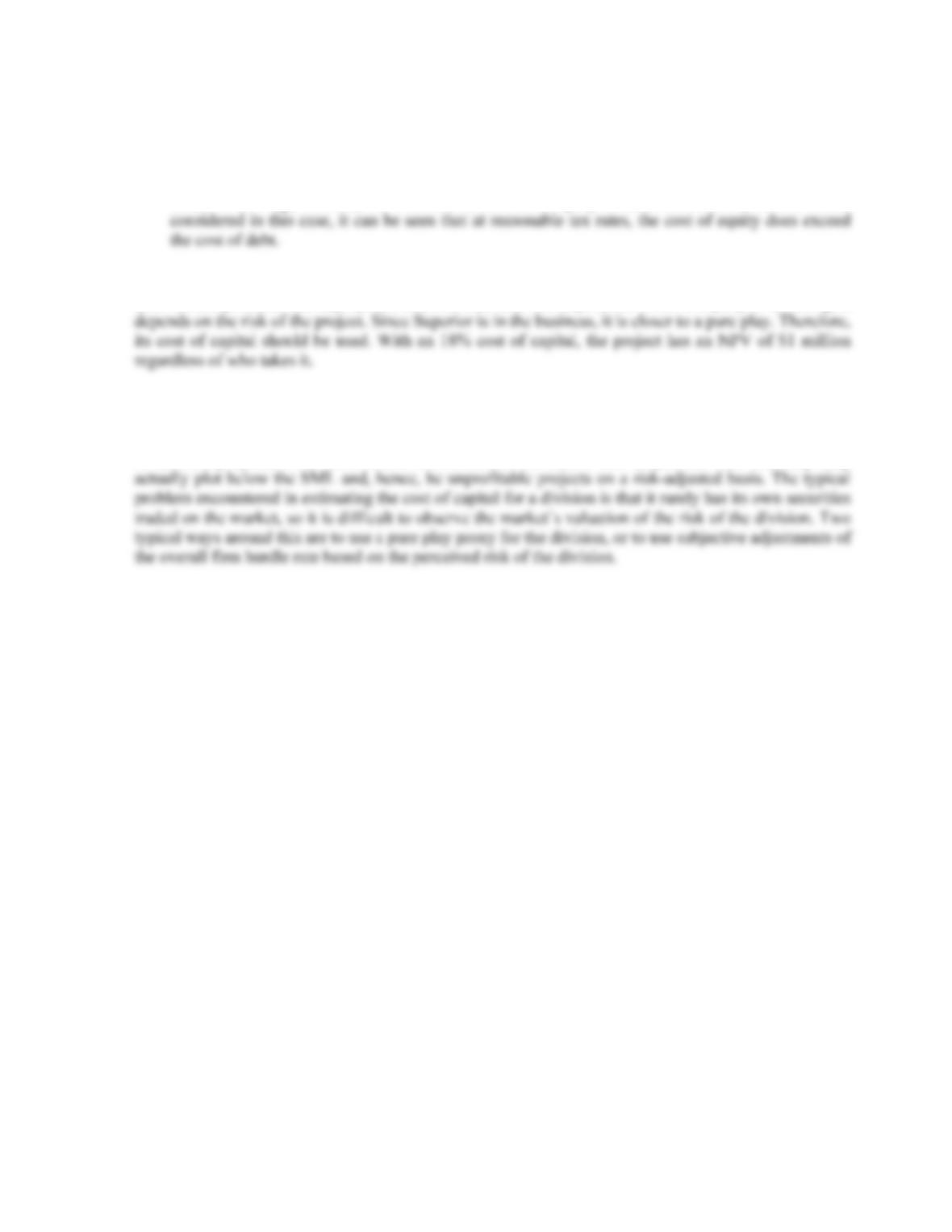Type
Quiz
Book Title
Fundamentals of Corporate Finance Standard Edition 9th Edition
ISBN 13
978-0073382395

### 978-0073382395 Chapter 14 Concepts Review and Critical Thinking Questions

April 3, 2019CHAPTER 14
COST OF CAPITAL
Answers to Concepts Review and Critical Thinking Questions
1. It is the minimum rate of return the firm must earn overall on its existing assets. If it earns more than
this, value is created.
2. Book values for debt are likely to be much closer to market values than are equity book values.
3. No. The cost of capital depends on the risk of the project, not the source of the money.
4. Interest expense is tax-deductible. There is no difference between pretax and aftertax equity costs.
5. The primary advantage of the DCF model is its simplicity. The method is disadvantaged in that (1) the
model is applicable only to firms that actually pay dividends; many do not; (2) even if a firm does pay
dividends, the DCF model requires a constant dividend growth rate forever; (3) the estimated cost of
equity from this method is very sensitive to changes in g, which is a very uncertain parameter; and (4)
6. Two primary advantages of the SML approach are that the model explicitly incorporates the relevant
risk of the stock and the method is more widely applicable than is the dividend discount model model,
since the SML doesn’t make any assumptions about the firm’s dividends. The primary disadvantages of
the SML method are (1) three parameters (the risk-free rate, the expected return on the market, and beta)
7. The appropriate aftertax cost of debt to the company is the interest rate it would have to pay if it were to
issue new debt today. Hence, if the YTM on outstanding bonds of the company is observed, the
company has an accurate estimate of its cost of debt. If the debt is privately-placed, the firm could still
estimate its cost of debt by (1) looking at the cost of debt for similar firms in similar risk classes, (2)B-252 SOLUTIONS
8. a. This only considers the dividend yield component of the required return on equity.
b. This is the current yield only, not the promised yield to maturity. In addition, it is based on the
book value of the liability, and it ignores taxes.
c. Equity is inherently more risky than debt (except, perhaps, in the unusual case where a firm’s
assets have a negative beta). For this reason, the cost of equity exceeds the cost of debt. If taxes are
9. R
Sup = .12 + .75(.08) = .1800 or 18.00%
Both should proceed. The appropriate discount rate does not depend on which company is investing; it
10. If the different operating divisions were in much different risk classes, then separate cost of capital
figures should be used for the different divisions; the use of a single, overall cost of capital would be
inappropriate. If the single hurdle rate were used, riskier divisions would tend to receive more funds for
investment projects, since their return would exceed the hurdle rate despite the fact that they may
Solutions to Questions and Problems
NOTE: All end of chapter problems were solved using a spreadsheet. Many problems require multiple steps.
Due to space and readability constraints, when these intermediate steps are included in this solutions
manual, rounding may appear to have occurred. However, the final answer for each problem is found
without rounding during any step in the problem.
Basic
1. With the information given, we can find the cost of equity using the dividend growth model. Using this
model, the cost of equity is:
R
E = [\$2.40(1.055)/\$52] + .055 = .1037 or 10.37%
2. Here we have information to calculate the cost of equity using the CAPM. The cost of equity is:
RE = .053 + 1.05(.12 – .053) = .1234 or 12.34%
3. We have the information available to calculate the cost of equity using the CAPM and the dividend
growth model. Using the CAPM, we find:
RE = .05 + 0.85(.08) = .1180 or 11.80%